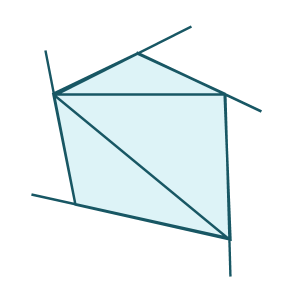Games
Problems
Go Pro!

9th grader Akhil from Kerala asks the following: "The sides of a quadrilateral are extended to form exterior angles. Find the sum of these exterior angles."

Hi Akhil, instead of just answering the question you asked, I'd like to explore the question in a little more depth, and explain why the answer is what it is. After all, if I just told you the answer, that would make for a pretty boring blog post!

So let's start off with a quadrilateral. I'm going to show you a picture of one below, and ask a question about it. How many degrees are there in the measures of all the interior angles of the quadrilateral?Now, you might already know the answer to that question; the sum of all the interior angles is 360 degrees. But can you prove that to be true? It's actually not hard to understand why that quadrilateral (and all quadrilaterals, for that matter) have an interior angle sum of 360 degrees. To see why, just look at this picture, in which I've divided the quadrilateral into triangles:How many triangles did it take to divide up that quadrilateral? It took two, right? And how many degrees does each of those triangles have? 180 degrees. And so altogether, how many degrees do they have in total? 180 x 2 = 360 degrees.

Understanding that the total of the interior angles is 360 degrees is important to finding the number of degrees in the exterior angles. Here's a picture that shows what you described - the sides being extended to form exterior angles:By extending these sides, we've created linear pairs - pairs of angles that form a straight line. Each linear pair includes an interior angle and an exterior angle. Each linear pair adds to 180 degrees (a straight line), and there are four linear pairs (because there are four sides).

So if there are 4 linear pairs, and each linear pair is 180 degrees, that's a total of 720 degrees. But 360 degrees of that is interior angles, so the exterior angles have to add up to 720 - 360 = 360 degrees! And that's your answer.

Now, it might be tempting to think that the interior angles of a polygon and the exterior angles of a polygon will always match, since they do in the case of a quadrilateral, but that is not the case. Let's take a look at a pentagon to see why. Here I'll give you a pentagon divided into triangles, and with sides extended:In the case of a pentagon, we can divide the polygon into 3 triangles, which means the interior angles add to 3 x 180 = 540 degrees.

We've created 5 linear pairs, which total 5 x 180 = 900 degrees.

Since there are 900 degrees total, and the interior angles add to 540 degrees, the sum of the exterior angles is 900 - 540 = 360 degrees!

It turns out that the sum of the exterior angles is 360 degrees regardless of whether it's a quadrilateral or a pentagon.

What do you think...can you generalize it even further? How many degrees are there in the interior of an n-gon? And how many degrees in the exterior angles of an n-gon? I'll leave it to you to figure that out. Thanks for asking, Akhil!# Blogs on This SiteReviews and book lists - books we love!The site administrator fields questions from visitors.Like us on Facebook to get updates about new resources